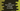# Java program to find the total count of words in a string### Java program to find the total count of words in a string :

In this tutorial, we will learn how to count the total number of words in a string in Java. The user will enter one string. Our program will count the total number of words in the string and print out the result.

Let’s take a look at the program first :

### Java Example program to find the count of words:

``````import java.util.Scanner;

public class Main {

//5
private static int countWords(String input){
//6
if(input == null)
return 1;

//7
String[] words = input.split("\\s+");

//8
return words.length;
}

public static void main(String[] args) {
//1
Scanner scanner = new Scanner(System.in);

//2
System.out.println("Enter a string to count number of words : ");

//3
String inputStr = scanner.nextLine();

//4
System.out.println("Total number of words in the string : "+countWords(inputStr));

}

}
``````

### Explanation :

The commented numbers in the above program denote the step-number below :

1. Create one Scanner object to read the inputs of the user.

2. Ask the user to enter a string.

3. Save the user-input string in variable inputStr.

4. Now, calculate the total number of words in the string. We are using one different method to find out the count.

5. private static int countWords(String input) method is used to find the count of words in a String. It takes one String as input and returns the total number of words in that input string.

6. Inside the method, first, we are checking if the input string is null if null return 1.

7. Split the given string by space. It returns an array of string. Save it in a string array. To split the string, we are using split() method. \s+ means it will split all words of that string separated by a single or multiple spaces.

8. Finally, return the size of the variable words or count of words in that string. Print out the result.

### Sample Output :

``````Enter a string to count number of words :
This is a sample String
Total number of words in the string : 5

Enter a string to count number of words :
Hello World
Total number of words in the string : 2

Enter a string to count number of words :
Hi
Total number of words in the string : 1

Enter a string to count number of words :
Once upon a time
Total number of words in the string : 4
``````